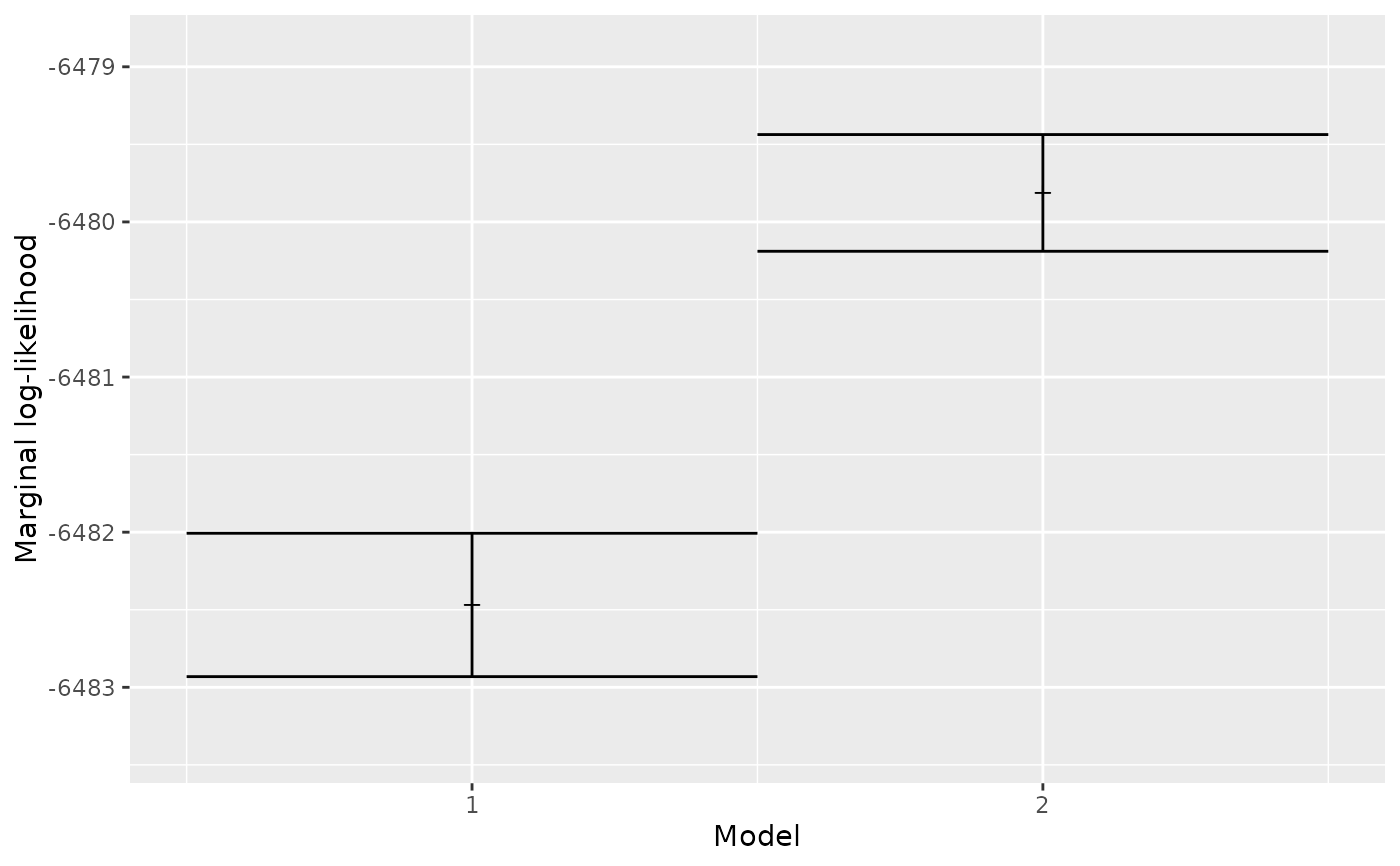Plot the `marg_liks`

## Usage

``plot_marg_liks(marg_liks)``

## Arguments

marg_liks

a table of (estimated) marginal likelihoods, as, for example, created by est_marg_liks. This data.frame has the following columns:

• `site_model_name`: name of the site model, must be an element of get_site_model_names

• `clock_model_name`: name of the clock model, must be an element of get_clock_model_names

• `tree_prior_name`: name of the tree prior, must be an element of get_tree_prior_names

• `marg_log_lik`: estimated marginal (natural) log likelihood

• `marg_log_lik_sd`: estimated error of `marg_log_lik`

• `weight`: relative model weight, a value from 1.0 (all evidence is in favor of this model combination) to 0.0 (no evidence in favor of this model combination)

• `ess`: effective sample size of the marginal likelihood estimation

Use get_test_marg_liks to get a test `marg_liks`. Use is_marg_liks to determine if a `marg_liks` is valid. Use check_marg_liks to check that a `marg_liks` is valid.

## Examples

``````plot_marg_liks(get_test_marg_liks())beastier::remove_beaustier_folders()
beastier::check_empty_beaustier_folders()
``````